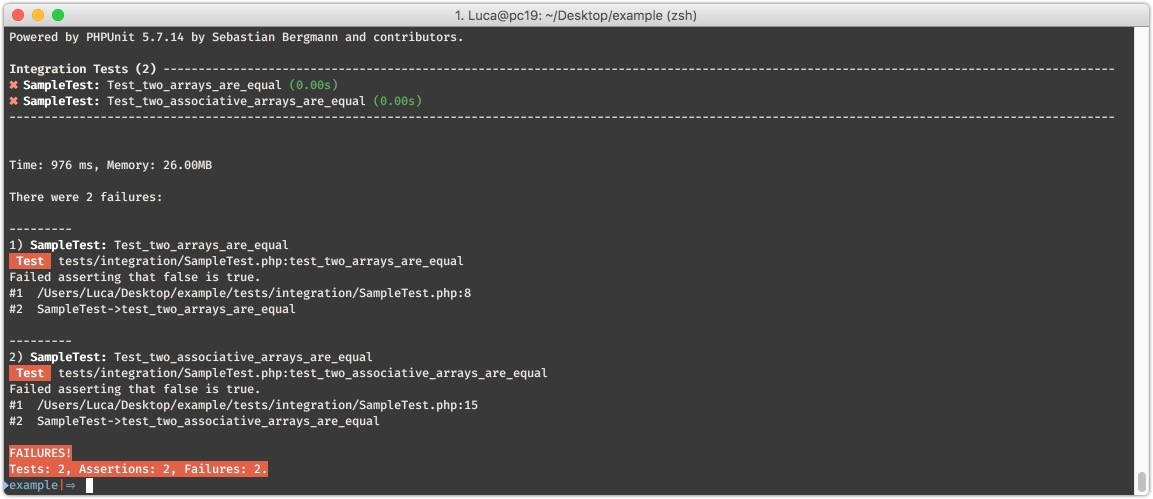# Four easy pieces about equality assertions in tests

Not all Equals are born equal.

## The basic block of testing

If I had to come up with a number I’d say 75% of my testing assertions are equality comparisons.
In the context of PhpUnit, Codeception and wp-browser tests this means code like this is my bread and butter:

public function test_I_get_what_I_expect(){
$value = 23;$expected = 11;

$object = new Worker();$got = $worker->workOn($value);

$this->assertEquals($expected, $got); } This is easy enough to read and understand but many developers get assertions, and use assertions, wrong leading to false successes or failures. ## Comparing arrays The “gotchas” of equality comparison become apparent when working with arrays: sometimes in unexpected ways; one of the two tests below will fail: public function test_two_arrays_are_equal(){$this->assertEquals(['foo','bar'], ['bar','foo']);
}

public function test_two_associtive_arrays_are_equal(){
$this->assertEquals(['lorem' => 'ipsum','dolor' => 'sit'], ['dolor' => 'sit','lorem' => 'ipsum']); }While both are compared in reverse order only the first test fails, to make things works I’m using the assertSame assertion: public function test_two_arrays_are_equal(){$this->assertSame(['foo','bar'], ['bar','foo']);
}

public function test_two_associtive_arrays_are_equal(){
$this->assertSame(['lorem' => 'ipsum','dolor' => 'sit'], ['dolor' => 'sit','lorem' => 'ipsum']); }Now both test fail. The reason behind the failures becomes easy to understand modifying the assertions into assertTrue ones: public function test_two_arrays_are_equal(){$this->assertTrue(['foo','bar'] == ['bar','foo']);
}

public function test_two_associtive_arrays_are_equal(){
$this->assertTrue(['lorem' => 'ipsum','dolor' => 'sit'] == ['dolor' => 'sit','lorem' => 'ipsum']); }And again: public function test_two_arrays_are_equal(){$this->assertTrue(['foo','bar'] === ['bar','foo']);
}

public function test_two_associtive_arrays_are_equal(){
$this->assertTrue(['lorem' => 'ipsum','dolor' => 'sit'] === ['dolor' => 'sit','lorem' => 'ipsum']); }## The underlying logic So the logic here is: • assertEquals is a loose comparison like == • assertSame is strict comparison like === The reason['foo','bar'] != ['bar','foo'] is that the two arrays can be rewritten like: $one = [
0 => 'foo',
1 => 'bar'
];
$two = [ 0 => 'bar' 1 => 'foo', ]; So: do the two arrays have the same key and value couples no matter the order? They don't. Using assertSame simply takes the order too into account. To compare two non associative arrays no matter the order one would need to sort them first: php public function test_two_arrays_are_equal() {$one = [ 'foo', 'bar' ];
$two = [ 'bar', 'foo' ]; sort($one );
sort( $two );$this->assertEquals( $one,$two );
}## WordPress specifics

Should I find myself testing for comparisons in a WordPress context I will tap into a neat method provided by [WordPress own PHPUnit powered testing suite](!g WordPress automated tests phpunit):

public function test_two_arrays_are_equal() {
$one = [ 'foo', 'bar' ];$two = [ 'bar', 'foo' ];
$this->assertEqualSets($one, \$two );
}`The function will sort the two array elements for me saving some lines of code.

## What should I use then?

Given the possibilities and limits of each comparison assertion I always use the loosest possible comparison given the subject return value requirements.
Do I really want to assert two things are the same? Maybe similar is enough?
Thinking about what comparison assertion is to be used when forces me to think about the subject under test and the contract it makes with its clients: is it granting an exact match or a loose one? Are the consuming client assuming an exact match or a close one?
An object providing a ranking should consistently provide fixed order and values while an object reporting about asynchronous jobs could provide a list of what was done without guaranteeing the order.